International
Tables for
Crystallography
Volume F
Crystallography of biological macromolecules
Edited by E. Arnold, D. M. Himmel and M. G. Rossmann

International Tables for Crystallography (2012). Vol. F, ch. 2.2, pp. 71-73

## Section 2.2.9. Quality indicators for the refined model

H. M. Einspahra* and M. S. Weissb

aPO Box 6483, Lawrenceville, NJ 08648–0483, United States, and bHelmholtz-Zentrum Berlin für Materialien und Energie, Macromolecular Crystallography (HZB-MX), Albert-Einstein-Str. 15, D-12489 Berlin, Germany
Correspondence e-mail:  hmeinspahr@yahoo.com

### 2.2.9. Quality indicators for the refined model

| top | pdf |

In MX, the observable-to-parameter ratio is mostly unfavourable. Therefore, structure refinements are carried out with boundary conditions, constraints and restraints. Constraints reduce the number of parameters which need to be refined, while restraints provide additional information to the refinement procedure that increases the number of observables. A refined model, therefore, has to fulfil not only the criterion that the crystallographic R factor [see equation (2.2.8.1)] is good and that the model fits well to the electron density, but also that it fits well to the restraints used in the refinement procedure.

Real-space residual, RSR. The real-space residual, RSR (Jones et al., 1991), quantifies the discrepancies between the electron-density maps ρ1, calculated directly from a structural model, and ρ2, calculated from experimental data. RSR can take the form of a real-space R factor RSRF and of a real-space correlation coefficient RSCC.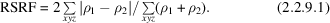The sum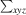runs over all grid points of the electron-density maps that are close to the model. A big advantage of RSRF is that is can be calculated on a residue-by-residue basis. It therefore gives a local picture of structure quality. It can also be used throughout model building and refinement in order to follow the improvement of the model locally on a per-residue basis.

RSCC is defined as the Pearson linear correlation coefficient [see equation (2.2.2.13)] between ρ1 and ρ2. Everything said about RSRF above applies to RSCC as well.

R.m.s. deviation from ideal of geometric parameter x. The root-mean-square deviation of a set of geometric parameters x from their ideal values is defined as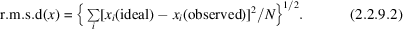The sum runs over all N instances of the geometric parameter occurring in a structure. The geometric parameters x that are typically considered are bond lengths, bond angles, dihedral angles, chiral volumes, planar groups etc. The ideal values for proteins are typically taken from the study of Engh & Huber (1991) and for nucleic acids from Parkinson et al. (1996).

Z score. A measure of the likelihood that an individual geo­metric parameter is correct is given by its Z score. The Z score is defined as the distance of an individual data point of a distribution from the mean of the distribution expressed in standard deviations. In the case described here, the mean values of the distribution are the ideal values taken from Engh & Huber (1991) and Parkinson et al. (1996).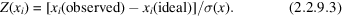Ideally, the Z score should be 0. A parameter that exhibits a Z score of less than −4 or greater than +4 is highly unlikely and calls for attention.

Root-mean-square Z score, r.m.s.-Z. Although r.m.s.d. values [see equation (2.2.9.2)] are still popular for use in judging the quality of refined macromolecular models, a much more useful statistic is the r.m.s. value of a distribution of Z scores or the r.m.s.-Z score.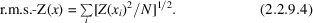The sum runs over all N instances of the geometric parameter x occurring in a structure. A very useful property of Z scores is that the r.m.s. values of Z-score distributions should always be 1. Significant deviations from the ideal value indicate potential problems. R.m.s. Z scores are widely used, for instance, in the program WHAT_CHECK (Hooft et al., 1996).

R.m.s.d. (NCS). The root-mean-square deviation from crystallographic symmetry between two molecules related by non­crystallographic symmetry (NCS) can be calculated from a superposition of the two molecules. It is defined as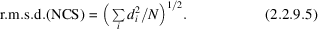The sum runs over N equivalent atom pairs with di being the distance between the two equivalent atoms after superposition.

### References

Engh, R. A. & Huber, R. (1991). Accurate bond and angle parameters for X-ray protein structure refinement. Acta Cryst. A47, 392–400.
Hooft, R. W. W., Vriend, G., Sander, C. & Abola, E. E. (1996). Errors in protein structures. Nature (London), 381, 272.
Jones, T. A., Zou, J.-Y., Cowan, S. W. & Kjeldgaard, M. (1991). Improved methods for building protein models in electron density maps and the location of errors in these models. Acta Cryst. A47, 110–119.
Parkinson, G., Vojtechovsky, J., Clowney, L., Brünger, A. T. & Berman, H. M. (1996). New parameters for the refinement of nucleic acid-containing structures. Acta Cryst. D52, 57–64.